Maple can be easily integrated into your development projects using a wide range of connectivity features. With code generation, external calling, the OpenMaple API, web connectivity, extensive import and export tools, and connectivity with other software, Maple can fit seamlessly into your toolchain.
New in Maple 16:

• Maple offers a technical computing solution that is tightly integrated with MATLAB®, providing direct access to all the commands, variables, and functions of each product while working in either environment. Important enhancements in Maple 16 include easier creation of matrices with symbolic entries, and support for the latest version of MATLAB®.
• Connectivity to CAD systems allows you to exchange parameter values and connect Maple calculations to a live CAD design. Maple 16 extends its support to the latest versions of major CAD systems: Siemens NX® 8, Autodesk Inventor® 2012, and Solidworks® 2012.
• Integration into the Symbolic Computation Software Composability Protocol (SCSCP) framework allows interoperability with special-purpose computer algebra systems.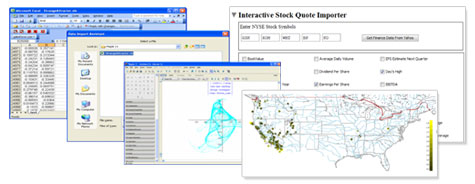MATLAB® Connectivity in Maple 16
Maple provides many different connectivity options with MATLAB®.
• Two-Way Integration between Maple and MATLAB®
This feature allows you to interact with both programs while they share the same variables and state. You have direct access to all the commands, variables, and functions of each product while working in either environment.
The MATLAB® lets you call on MATLAB® to perform calculations from the Maple environment, and return the results to Maple for further analysis.
• MATLAB® Code Generation
Maple can generate optimized MATLAB® code from Maple expressions and procedures.
• MATLAB® to Maple code translation
This facility helps you convert your existing MATLAB® code into Maple syntax. Use it for new projects, to expand existing projects, or simply to see how a command you know from MATLAB® is represented in Maple.

Example: Calling Maple from MATLAB®
Maple commands, packages, assistants, and even the whole user interface is accessible from MATLAB®. Using MATLAB® as your main interface you can call on Maple for symbolic computations by linking seamlessly to the Maple math engine.

The most basic symbolic object is a symbol. To start using Maple, create a sym object, x, in MATLAB®. More complicated expressions can then be formed using your declared symbolic variable, x.

>> x = sym('x')

x =

x

>> x^3+cos(2*x)-1

ans =

3
x + cos(2 x) - 1

Symbolic expressions and equations can then, among other things, be differentiated, integrated, factored, and solved exactly using MATLAB® front-ends to Maple commands.

>> diff(x^3)

ans =

2
3 x

>> int(3*x^2)

ans =

3
x

>> factor(x^4+10*x^3+35*x^2+50*x+24)

ans =

(x + 4) (x + 3) (x + 2) (x + 1)

>> expand(ans)

ans =

4   3   2
x + 10 x + 35 x + 50 x + 24

>> syms x y z
>> solve( 3*x+1*y+4*z-5, 8*x+19*y+11*z-94, x+y/4+z-11)

ans =

x: 39
y: 72/13
z: -382/13

New in Maple 16 is the ability to easily create symbolic matrices. The sym command now accepts options for specifying the size and format of a matrix to be filled with symbolic entries.

>> A = sym('A',[3 3])

A =

[A1_1    A1_2    A1_3]
[                    ]
[A2_1    A2_2    A2_3]
[                    ]
[A3_1    A3_2    A3_3]

The resulting matrices can be used to compute exact symbolic answers. For example, the determinant of the above matrix as an equation can be calculated like this:

>> det(A)

ans =

A1_1 A2_2 A3_3 - A1_1 A2_3 A3_2 + A2_1 A3_2 A1_3 - A2_1 A1_2 A3_3

+ A3_1 A1_2 A2_3 - A3_1 A2_2 A1_3

The format of the matrix entries can be customized using a string template. Standard operations like matrix multiplication are known to the package and overloaded accordingly.

>> B = sym('B%d%d',[2 3])

B =

[B11    B12    B13]
[                 ]
[B21    B22    B23]

>> B * A

ans =

[B11 A1_1 + B12 A2_1 + B13 A3_1 , B11 A1_2 + B12 A2_2 + B13 A3_2 ,

B11 A1_3 + B12 A2_3 + B13 A3_3]

[B21 A1_1 + B22 A2_1 + B23 A3_1 , B21 A1_2 + B22 A2_2 + B23 A3_2 ,

B21 A1_3 + B22 A2_3 + B23 A3_3]

The New SCSCP Package in Maple 16

MMaplesoft was one of the partner organizations involved in the research project SCIEnce (Symbolic Computation Infrastructure for Europe). This project aims to connect different powerful computer algebra systems, including Maple, via web services. The underlying communication protocol, SCSCP (Symbolic Computation software Composability Protocol), has been introduced in this project to facilitate the communication between different computer algebra systems such as GAP and KANT.

The SCSCP[Client] and SCSCP[Server] packages have been developed to support this protocol. The SCSCP[Server] package is a part of the Maple SCSCP server's implementation. MapleNet is required to run a Maple SCSCP server. MapleNet actively listens to incoming connections and calls SCSCP[Server] to carry out the computations. The SCSCP[Server] package also provides utilities to modify the web services provided.

Example: Connecting to the GAP computer algebra system

 >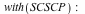>Let's see what is supported in a Maple SCSCP server.

 >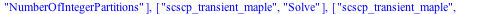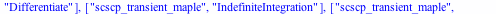>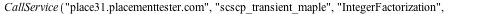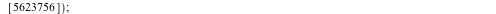The following shows how SCSCP[Client] package interacts with a remote server running GAP computer algebra system.

 >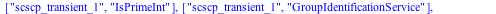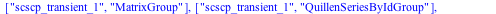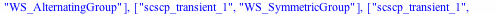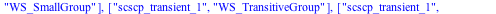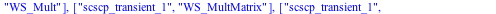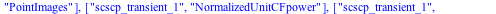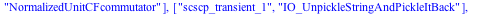>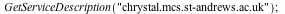>Find the library number of the group:

 >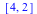Find the conjugacy classes of the group:

 >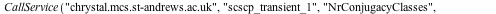How to Proceed: Pricing & Purchase Evaluate Upgrade Get Price Quote Buy Online